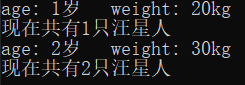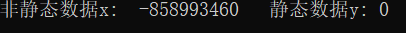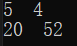# C++ 中 static 与 const 的用法及对比

## 一、类的静态成员

如果某个属性为整个类所共有，不属于任何一个具体对象，则采用 static 关键字来声明静态成员。

#### 使用方法----- "类名：：标识符“   （注意：static 数据成员的初始化要在类定义之外在加以定义）

1.静态数据成员

#include<iostream>
using namespace std;

class Dog {
public:
Dog(int age, int weight);
void show();
~Dog() {}
private:
static int number;
int age, weight;
};
int Dog::number = 0;        //static 数据的定义【因为需要这种方式专门为它们分配空间，而非静态数据是与它们的所属对象一同分配空间的】

Dog::Dog(int age, int weight) {　　　　//构造函数
this->age = age;
this->weight = weight;
number++;
}

void Dog::show() {
cout << "age: " << age << "岁   " << "weight: " << weight <<"kg"<< endl;
cout << "现在共有" << number << "只汪星人" << endl;
}

int main() {
Dog dog(1, 20);
dog.show();

Dog dog2(2, 30);
dog2.show();
return 0;
}    

结果如下：有个细节：static 数据类型 在定义时不给它设定值的化，系统默认为 0，而非静态数据类型则随机赋值。（ps:下面代码 14 行也值得注意）

 1 #include<iostream>
2 using namespace std;
3
4 class Point {
5 public:
6     Point() {};
7     void set(int x, int y);
8     int getx() { return x; }
9     int gety() { return y; }
10 private:
11     int x;
12     static int y;
13 };
14 int Point::y;            //切记，哪怕不给static类型 定义时赋初值，也需要在类外面定义【即分配空间】，否则会报错
15 void Point::set(int x, int y) {
16     this->x = x;
17     this->y = y;
18 }
19 int main() {
20     Point p;
21     cout << p.getx() << "   " << p.gety() << endl;
22     return 0;
23 }

结果如下：### 2.静态成员函数

静态成员函数可以直接访问该类的数据和函数成员，而访问非静态成员必须通过对象名
class A {
public:
static void f(A a);
private:
int x;
static int y;
};
static int y;
void A::f(A a) {
cout << x;          //错误
cout << a.x;        //正确

cout << y;　　　　　　//直接访问static 数据正确
}

从上面几个例子中，我们发现静态成员函数可以直接访问静态数据类型访问非静态数据类型时需要通过对象名来实现

 1 #include <iostream>
2
3 using namespace std;
4
5 class Cat {
6 public:
7     Cat() { numOfCats++; }
8
9     static void getNumOfCats();
10
11 private:
12     static int numOfCats;
13 };
14
15 int Cat::numOfCats = 0;
16
17 void Cat::getNumOfCats() {
18     cout << "猫的数量：" << numOfCats << endl;
19 }
20
21 int main() {
22     Cat::getNumOfCats();    //static函数属于类，并不属于某个对象，所以可以这样调用，当然通过对象也可以调用
23     Cat cat;
24     cat.getNumOfCats();
25     Cat cat2;
26     cat.getNumOfCats();
27 }

## 二. 常对象

常对象是这样的对象：它的数据成员值在对下岗的整个生存周期内不能被改变，也就是说，常对象必须进行初始化而且不能被更新！

语法形式：  const   类型说明符    对象名；

int main() {
const int n = 10;     //正确，用10对常对象初始化
n = 10;                //错误，常对象不能被赋值
}

用const 修饰的类成员

1. 常成员函数

类型说明符 函数命（参数表） const ；

注意：

（1）const 是函数类型的组成成分，因此在函数定义部分也要带const

（2）一个对象是常对象 ，则只能通过该常对象调用它的 常成员函数

（3）const关键字可以用于重载

 1 #include<iostream>
2 using namespace std;
3
4 class R {
5 public:
6     R(int r1, int r2) :r1(r1), r2(r2) {}
7     void print();
8     void print() const;                //函数重载
9 private:
10     int r1, r2;
11 };
12
13 void R::print() {
14     cout << r1 << "  " << r2 << endl;
15 }
16
17 void R::print()const {　　　　　　　　　　//定义时const 依旧是要带的
18     cout << r1 << "  " << r2 << endl;
19 }
20
21 int main() {
22     R a(5, 4);
23     a.print();　　　　　　　　　　　　　　//调用13行print函数
24     const R b(20, 52);
25     b.print();　　　　　　　　　　　　　　//调用17行print函数
26     return 0;
27 }在逐步调试的过程中发现 常对象 b 在调用print函数时，调用了常成员函数，而非const对象a调用了 非常成员函数

ps:如果该程序中只有带const的print函数 则 普通对象a也是调用有const的print函数

But ,常对象b不能调用 普通print函数，因为普通print函数有着改值的可能，但常对象b不能改变值

2.常数据成员

将上面的代码类的声明改为

class R {
public:
R(int r1, int r2) :r1(r1), r2(r2) {}
void print();
void print() const;
private:
const int r1, r2;        //改为常数据
};

class Dog {
public:
Dog() {}
private:
static const int a = 0;
};

//=========上下二者等价=================

class Dog {
public:
Dog(){}
private:
static const int a;
};
int Dog::a = 0;

# 总结：

1.  static 类型数据可以通过 static类型或者 非static类型函数访问并可以改变；

const类型数据不能改变;

2.  static 函数可以直接访问 类中 static 类型数据，但访问非static数据需通过对象访问；

const类型函数可以被调用于非const对象，但在对象是const类型时，通过该对象只能调用const类型函数

=============================================================================================

posted @ 2019-09-26 14:35  果冻小布丁  阅读(1419)  评论(0编辑  收藏  举报